# Tank full

2 hl of water were poured into a block-shaped tank with dimensions of 450 mm and 600 mm and a height of 900 mm. How many liters of water need to be added to keep the tank full?

x =  43 l

### Step-by-step explanation: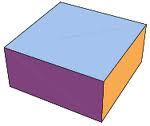Did you find an error or inaccuracy? Feel free to write us. Thank you!Tips to related online calculators
Do you know the volume and unit volume, and want to convert volume units?

## Related math problems and questions:

• Water tank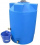The block-shaped tank is 2.5 m long, 100 cm wide and 12 dm high. In how many minutes will it be filled with water to two thirds if 40 liters of water flow into the tank per minute?
• Water tankWhat is the height of the cuboid-shaped tank with the bottom dimensions of 80 cm and 50 cm if the 480 liters of water reach 10 cm below the top?
• Swimming pool 4The pool shaped cuboid measuring 12.5 m × 640 cm at the bottom is 960hl water. To what height in meters reaches the water level?
• Swimming poolThe swimming pool has the shape of a block with dimensions of 70dm, 25m, 200cm. How many hl of water can fit into the pool?
• Water tank300hl of water was filled into the tank 12 m long and 6 m wide. How high does it reach?
• WaterInto a full cylindrical tank high 3 m with a base radius of 2.5 m, we insert cuboid with dimensions 1.7 m, 1.3 m, 1.9 m. How many liters of water will overflow out?
• Cylindrical tank9.6 hl of water is poured into a cylindrical tank with a bottom diameter of 1.2 m. What height in centimeters does the water reach?
• The tankThe tank is full up to 4/5 of the total height and contains 240 hl of water. The area of the base is 6 square meters. What is the height of the tank?
• Tank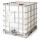How many minutes does it take to fill the tank to 25 cubic meters of water filled 150hl per hour?
• AquariumFind how many dm2 of glass we need to make a block-shaped aquarium (the top is not covered) if the dimensions of the aquarium are to be: width 50 cm, length 120 cm, and height 8.5 dm.
• Four prismsQuestion No. 1: The prism has the dimensions a = 2.5 cm, b = 100 mm, c = 12 cm. What is its volume? a) 3000 cm2 b) 300 cm2 c) 3000 cm3 d) 300 cm3 Question No.2: The prism base is a rhombus with a side length of 30 cm and a height of 27 cm. The height of t
• Snails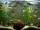How many liters of water will fit in an aquarium with bottom dimensions of 30 cm and 25 cm and a height of 60 cm, if we pour water up to a height of 58 cm? How many most snails can we keep in an aquarium, if we know that snails need 600 cm ^ 3 of water fo
• Empty barrel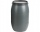In the backyard is an empty barrel with a volume of 2 hl. How many liters are missing at the edge, when 53 liters flowed into it for 1 day and 68 liters of water for 2 days?
• Water tankA 288 hectoliter of water was poured into the tank with dimensions 12 m and 6 m bottom and 2 m depth. What part of the volume of the tank water occupied? Calculate the surface of tank wetted with water.
• Fire tankHow deep is the fire tank with the dimensions of the bottom 7m and 12m, when filled with 420 m3 of water?
• HectolitersHow many hectoliters of water fits into cuboid tank with dimensions of a = 3.5 m b = 2.5 m c = 1.4 m?
• Annual rainfallThe average annual rainfall is 686 mm. How many liters will fall on the 1-hectare field?